# Introduction

Numeric multivariate data is ubiquitous and viewing data within data-space (rather than summarized as parameters or statistics) is a critical part of exploratory data analysis and the data analysis workflow in general. Viewing data that exists in more than 3 numeric dimensions quickly becomes complex. Linear projections of high dimensional spaces offer a scalable way to extend viewing these data-spaces as the dimension of the data increases. The dynamic viewing of many linear projections encompasses a class of techniques collectively known as tours.

The package spinifex allows the application of manual tours, where a selected variable is rotated fully into and out of the give projection basis. It’s also compatible with other tours from the tourr package and extends graphics display to plotly and gganimate.

## Dynamic projections of high dimensional data, tours

Tours are a class of dynamic orthogonal (linear) projections that embed $$p-$$dimensional (Euclidean) space into a $$d-$$dimensional subspace and animates many such projections as the projection basis (orientation) is rotated. Tours are useful in identify clustering, outliers, and structure held within numeric multivariate data.

This concept is illustrated well with the anecdote of shadow puppets. Suppose a bar stool is held in front of a light source. This is a linear projection of a 3D object (bar stool) down to 2D (its shadow). If we observe the shadow change over time as the bar stool is rotated, we are watching a 3- to 2D tour. Some projections may not convey much information (the seat may only cast a circular shadow), but as we watch the stool rotate our visual interpretation quickly understands the geometry of the object. The same is true for tours, each view holds some information, and a better understanding of the structure is gained over time.

We focus on the application of the manual tours in this document. In a manual tour, the contributions of one variable are manipulated to show the impact that it has on the structure of the projection. Controlling the coefficients of a single variable can be insightful after finding a projection of interest, perhaps with the use of a guided tour. A wider application of tours can be accomplished with with the package tourr, CRAN.R-project.org/package=tourr.

## Notation

• n - the number of observations in the data
• p - the number of variables/dimensions in the data
• d - number of dimensions in the projection, $$d \leq p$$, typically 2
• basis - $$[p,~d]$$ orthonormal matrix, orientation of the variables projecting from $$p-$$ to $$d-$$space
• reference axes/frame - graphical display of the basis, line segments on a unit circle, showing how each variable contributes to the projection space
• manip var - the selected variable to manipulate into and out of the projection, highlighted in blue below

# Usage

In the below examples we go through some use cases for manual tours. To get started we’ll load a couple packages we use.

library("tourr")
library("spinifex")
library("ggplot2")
library("dplyr")

## Example 1 - Rotate an arbitrary basis (& utility functions)

For this example, we’ll be using the flea data set. It consists of 74 observations of flea beetles across 6 numeric variables and a categorical variable of the species of flea beetle (with 3 levels).

The following example will explore how changing the contributions of the variable, head, will affect the structure of the projection. We’ll initialize a random basis and then view the manipulation space.

dat_std <- scale_sd(tourr::flea[,-7])
bas_pca <- basis_pca(dat_std)
clas <- tourr::flea[, 7]

ggtour(basis_array = bas_pca, data = dat_std) +
proto_default(aes_args = list(color = clas))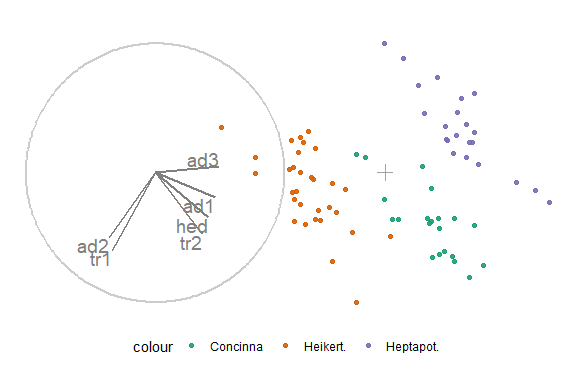We started from a random basis, that explains the orthogonal projection from $$p$$ to $$d$$ space. Use view_frame() to see the reference axes, a visual depiction of how the variables contributed to the xy directions of the 2D projection.

We want to explore how the coefficients of aede2 contribute to the structure in this projection, so we set the manip_var to its column number, 3. In order to change the contributions without breaking the orthogonality of the other variables, we need to add a dimension. We call this new space the manipulation space, which can be viewed with the function view_manip_space(). Note that the projection plane containing the reference frame is laid down on the surface, while the manipulation dimension is at a right angle out-of-plane, with a full contribution on the manip var.

view_manip_space(basis = bas_pca, manip_var = 3)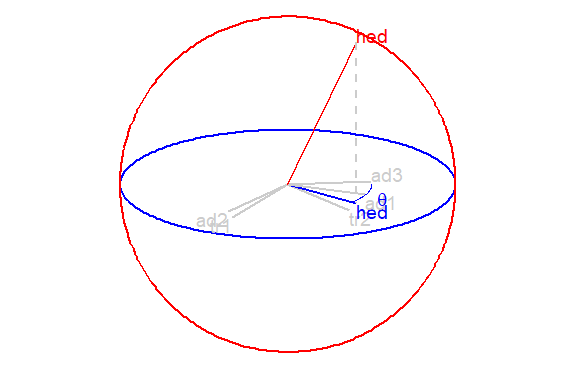Now we have the freedom to change the contributions of aede2, we do so by controlling the values of the in-plane angle, $$\theta$$, and the out of plane angle, $$\phi$$. in this example we’ll perform a radial manual tour, holding $$\theta$$ constant while we vary the values of $$\phi$$ to remove and maximize the contribution of the manip var.

mt_path <- manual_tour(basis = bas_pca, manip_var = 3)

my_ggtour <- ggtour(basis_array = mt_path, data = dat_std) +
proto_default(aes_args = list(color = clas, shape = clas))
animate_plotly(ggtour = my_ggtour)

plotly animation not rendered in this vignette, but will display in viewer

## Example 2 - Rotating a glyph map

Another of the manual tour is the creation a glyph-maps, where time series can be shown side-by-side, like faceting (offsetting) on their lat/long physical positions. We’ll use the following GGally glyph-map as an example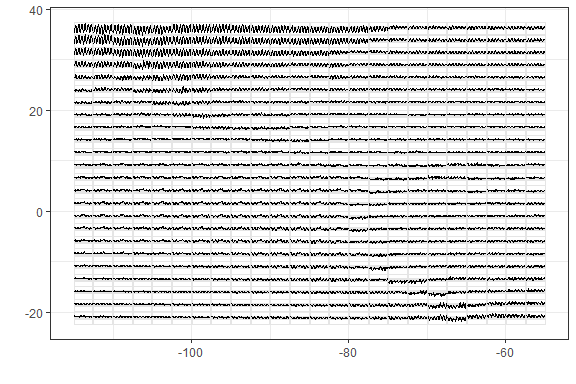We’ll perform a horizontal rotation and followed by the vertical rotation before bringing them together. we apply the horizontal rotation ($$\theta$$= 0) on the day values (manip_var = 3). Pre-multiply the data with the rotation matrix to project the data.

## Initialize
nasa_std <- cbind(
GGally::nasa[c("x", "y")],
scale_sd(GGally::nasa[c("day", "surftemp")])
)
bas <- tourr::basis_init(ncol(nasa_std), 2)

## Horizontal rotation
m_sp_x    <- create_manip_space(basis = bas, manip_var = 3)
rot_mat_x <- rotate_manip_space(manip_space = m_sp_x,
theta = 0, phi = pi / 6)
rot_x     <- data.frame(as.matrix(nasa_std) %*% as.matrix(rot_mat_x))
colnames(rot_x) <- c("x1", "x2", "x_manip_sp")

Likewise, we’ll perform a vertical rotation ($$\theta$$= pi/2) on surface temperature (manip_var = 4). Combine the rotations and plot just the rotated values without lat/long or x/y markers, neat!

## Vertical rotation
m_sp_y    <- create_manip_space(basis = bas, manip_var = 4)
rot_mat_y <- rotate_manip_space(manip_space = m_sp_y,
theta = pi / 2, phi = pi / 6)
rot_y     <- data.frame(as.matrix(nasa_std) %*% as.matrix(rot_mat_y))
colnames(rot_y) <- c("y1", "y2", "y_manip_sp")

## Combine rotations
rot_xy <- bind_cols(rot_x, rot_y, .name_repair = "unique") %>%
select(x = x1, y = y2)

ggplot(rot_xy, aes(x = x, y = y)) + geom_point(size = 0.3) +
theme_bw() + labs(x = "", y = "")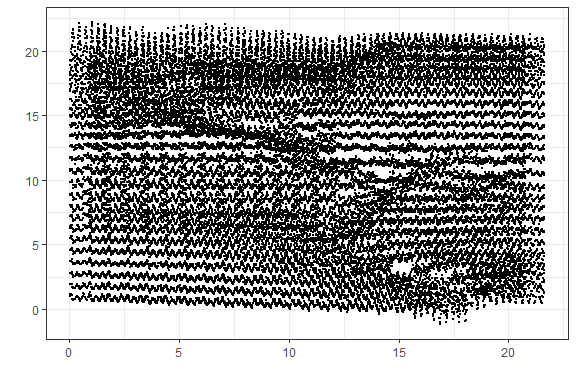## Example 3 - Rotation after holes guided tour

Radial tours are often employed after an interesting feature has been identified. How the contributions of each variable impact the structure of resulting PCA, for example, might be interesting to explore. Alternatively, we can perform projection pursuit to maximize an objective function within the projection and performing a hill-climbing algorithm and explore what effect the contributions of the variables have there. Let’s do just that, optimizing a Holes indexed guided tour using the package tourr.

dat_std <- scale_sd(tourr::flea[, 1:6])
holes_path <- tourr::save_history(dat_std, tourr::guided_tour(tourr::holes(), ))

ggt <- ggtour(holes_path, dat_std) + proto_default()
animate_plotly(ggt)

This shows the optimization path of the holes index. We’ll grab the reference axes from the last frame and used that a starting orientation for a manual tour.

## We added the basis_* functions, including basis_guided() to help:
holes_bas <- basis_guided(dat_std, index_f = tourr::holes(), d = 2)

ggtour(holes_bas, dat_std) +
proto_default(aes_args = list(color = clas, shape = clas))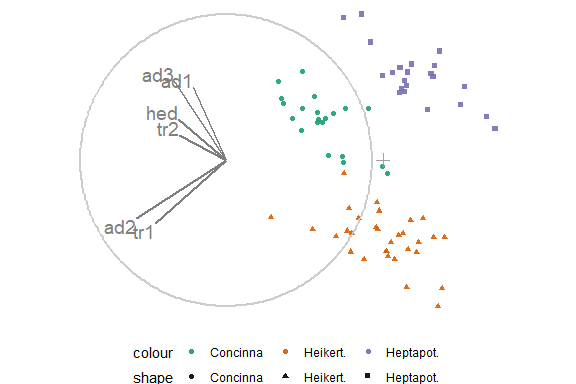We’ll select aede2 as the manip_var, as it is mostly orthogonal to four of the other variables and often has a larger contribution than tars1.

## Alternatively, ask for the variable by rank of the magnitude in the basis:
(mv <- manip_var_of(holes_bas, rank = 1))
mt_path <- manual_tour(holes_bas, mv)
ggt <- ggtour(mt_path, dat_std) +
proto_default(aes_args = list(color = clas, shape = clas))
animate_plotly(ggt)

We can see that aede2 is important in distinguishing between the purple cluster and the green cluster. However, even when its contribution is zeros the contribution of tars1 is enough to keep the cluster from overlapping.

# Summary

Dynamic linear projection of numeric data, collectively known as $$tours$$ is an important tool for visualizing data space as dimensionality increases. This package, spinifex, extends the tourr package and allows for manual tours. It also allows tours to be rendered by the animation packages plotly and gganimate.

## Namesake

The name ‘spinifex’ comes from the spinifex hopping mouse (wiki), a nocturnal dessert mouse common to arid zones in central and western Australia. As to its relation to this work, in the words of Di Cook “It spins, it hops and needs a ‘mouse’.”

## Acknowledgements

This package and vignette were made in R with much support from devtools and roxygen2.

Thanks to Prof. Dianne Cook for providing focus, namesake, and the groundwork underpinning this work. Thanks to Dr. Ursula Laa for use-cases and applications.